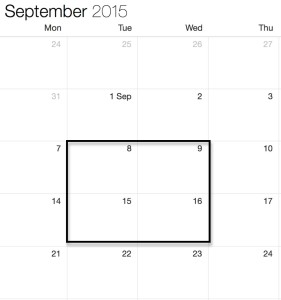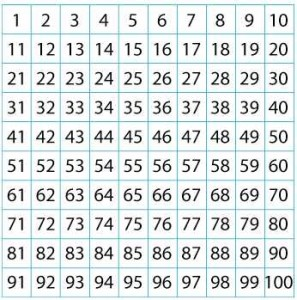On a calendar put a box around four numbers.Add the numbers in opposite corners.
For example 8+16 and 9+15.
What do you notice about the answers?

Put a box around another set of four numbers and try this again.
What happens and why?
Try multiplying – perhaps you could use a calculator to help.

What happens in other months?

Do the same with a hundred square.Again put a box around four numbers. Add the numbers in opposite corners.
What do you notice about the answers? Explain why this happens.

Try multiplying and explain what happens.

Teacher Notes CALENDAR PATTERNS

For a variation on this activity see NRICH

Tagged with: